+4

# Machine Learning: K-Means Clustering

Hello everyone, welcome to another post on machine learning. This time I'm going to discuss the unsupervised approach of machine learning. As discussed briefly in the previous post, unsupervised learning technique involves data that do not have any label on them. What it means, in technical terms, is that, we have a dataset and we want to identify which piece of the data belongs to which class or group by dividing them into smaller substes.

Take a collection of photos of 10 of your friends for example. You want to sort them out and group them together in 10 batches each containing photos of only one of your friends. What you need to do is divide the photos in 10 separate groups for the 10 of your friends. You do this based on their facial features that you very well know of. If you want a mindless thing (computer, software or machine) to get this job done for you, you will need an approach in which that thing will know nothing about which photo belongs to which friend, but, based on their facial features, it will categorize and group them together, just like Picasa did in its glorious days and Facebook is doing right now for tag suggestion. A clustering algorithm will come in handy in such a scenario.

As the name suggests, a clustering algorithm makes clusters of unlabeled data that you feed to it. Take a look below where the data is plotted but we cannot tell which one of them belongs to which class: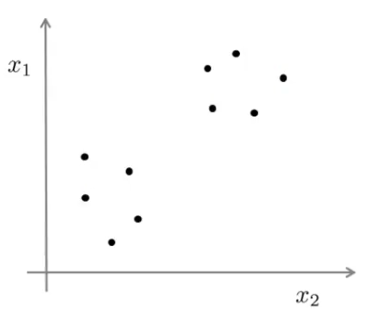Our clustering algorithm will take things in hand, do all the dirty jobs of computing and give you this: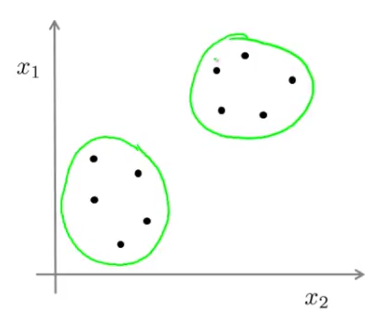As you can see, the data is now clustered in two separate classes as indicated by the green circle around them.

There are a lot more applications of clustering algorithms than I can think of. It's used in market segmentation in order to manufacture products for target market segments, social network analysis to identify coherent groups of people that you can manipulate (just kidding), astronomical data analysis from the images taken by the space telescopes to categorize different types of stars, stages of star formation or find out habitable planets like they did very recently, that we may one day live on.

There is a bunch of skilled players in the arena of the game of clustering. K-means, Fuzzy C-means, Hierarchical clustering, Mixture of Gaussian etc. are some of the mouthful names of these players. Among them, the K-means is the most prolific, that is, the most widely used clustering algorithm out there. This post is all about this simple, yet powerful clustering method.

# K-Means Algorithm

## A Simple Graphical Illustration

The K-means clustering algorithm is probably best explained in pictures. Have a look below where all the training examples of our dataset are plotted in green dots: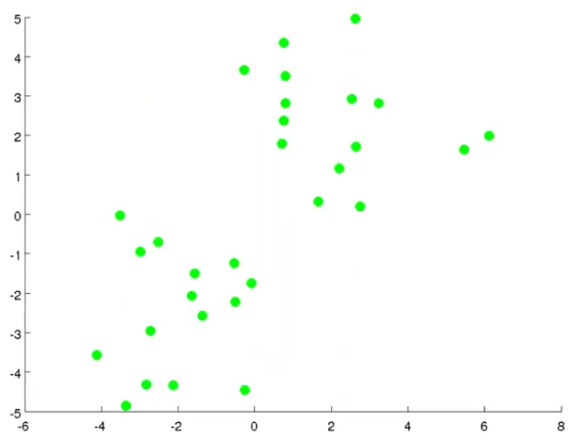Suppose we want to group the data into two clusters. To do that, the first thing we need is randomly generate two points called cluster centroids as shown in the red and blue cross (x) signs: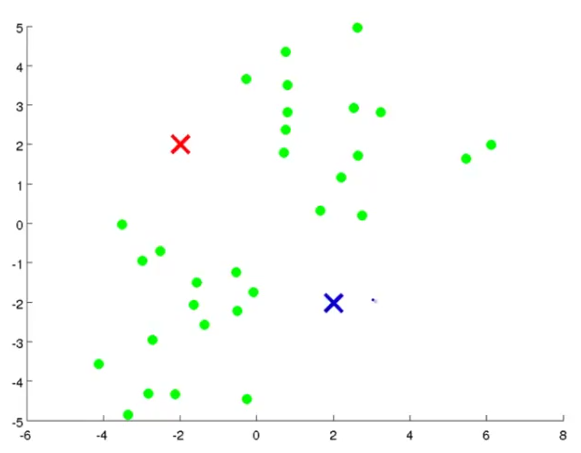Then the first step we'll do is called cluster assignment. That is, we will iterate through a loop to get all the example data and assign the data points to each of these two cluster centroids. We do that by figuring out which of the cluster centroids is closer to each data points. So, the figure will look like this: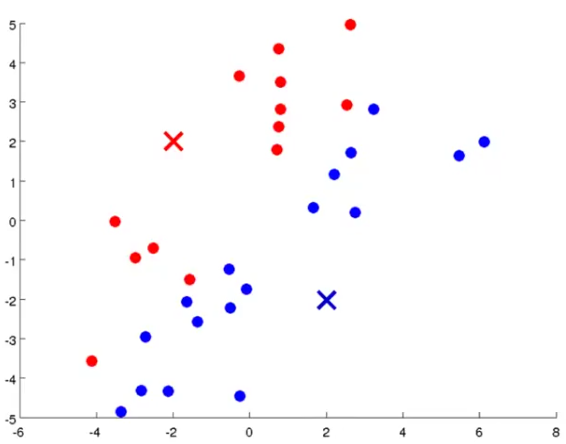The other step of the K-means is called move centroid. In this step, we will iterate through another loop to move each of the cluster centroids to the mean/average of the data points that are assigned to them. After this step, the figure will look like the following: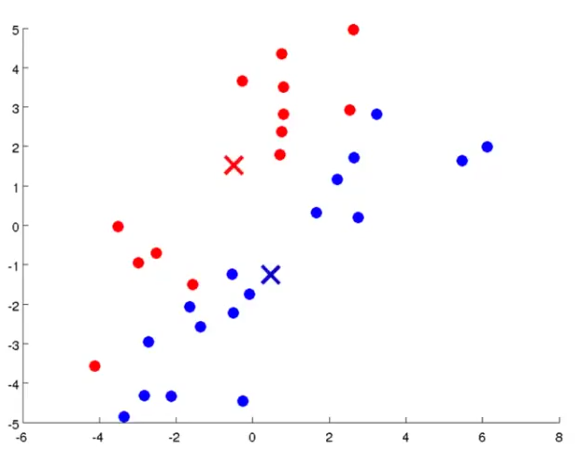Now, we will repeat the cluster assignment step once again so that the figure will look like this:Now do the same for the move centroid step and you will get this: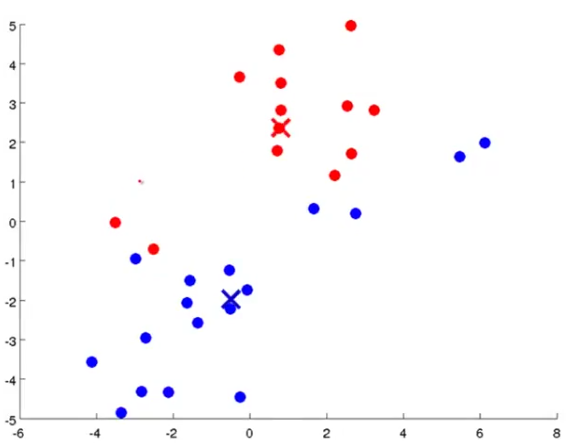If we repeat the two steps once again, then we will get the following respectively:Cluster assignment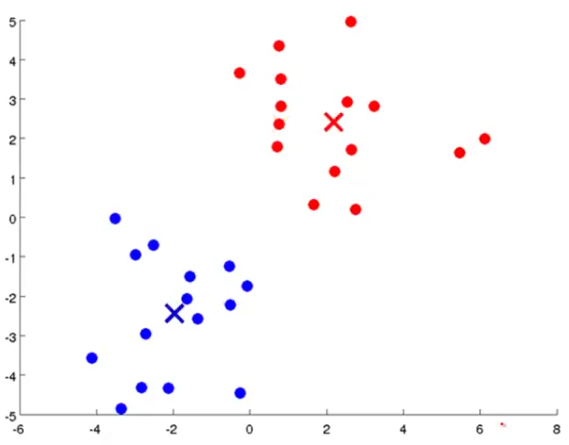Move centroids

At this point we are pretty much done. If we repeat the steps further, the centroids won't move very much and the colors of the data points won't change. So, this is the cluster that we are looking for. Simple, isn't it?

## The Algorithm

Here is the K-means algorithm in mathematical terms, if you're interested (I know you are).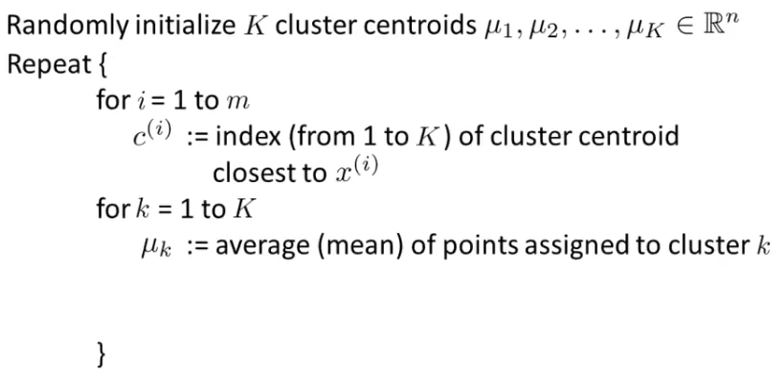Here, K is the number of clusters that we want to divide our dataset into and x is the feature vector of m training examples. μ1, μ2 etc. are the cluster centroids up to μK.

Within the outermost loop, there are two loops where we do the steps of cluster assignment and moving centroids. In cluster assignment, we get the index of the centroid which is the closest to a given example x. In the second loop we move the cluster centroid to the mean of its assigned data for all the K clusters.

## Cost Function

If you've read my previous article, you should be familiar with this term by now. The cost function of our K-means clustering will tell us how good it is in creating clusters, because, sometimes K-means can give us disastrous results like shown below: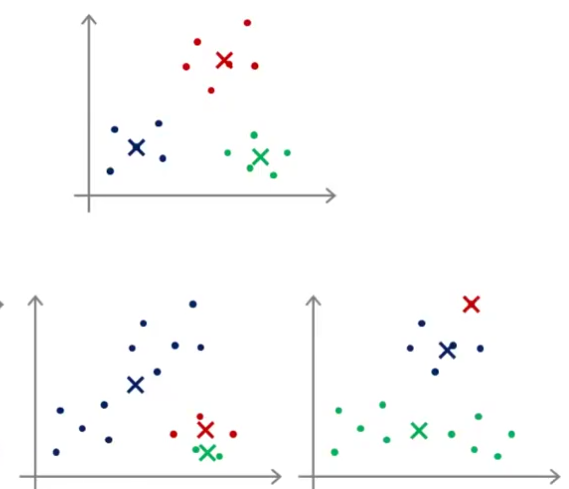To overcome this, first we need a cost function that will look like the following: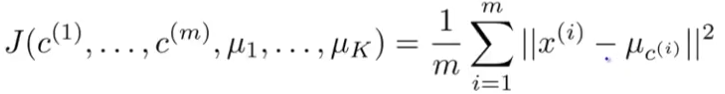Here,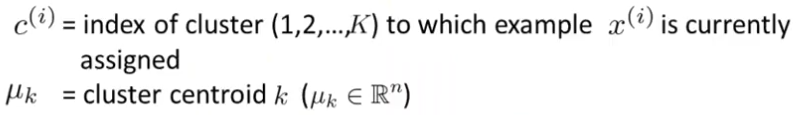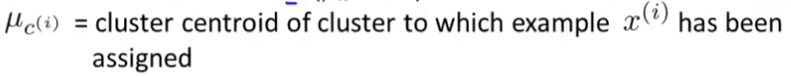As you can see, the cost function, also known as the distortion function, gives us the mean of the squared distance of all the data points to its corresponding cluster centroid. So, if we make a bunch of clusters, the one with the lowest cost is the one we should choose from them. In mathematical terms: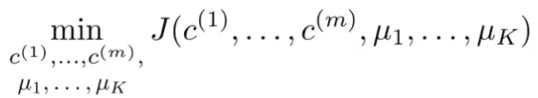## Random Initialization

As we've seen, we have to randomly initialize the cluster centroids for K number of times. Now the question arises - how do we choose the value of K? The simplest answer to this question can be summarized as follows:

1. Let K < m where m is the number of training examples.
2. Randomly choose K examples from the total of m
3. Set μ1,...,μK equal to these K examples.

Now, run K-means and compute the cost function. Then do the above steps again and run K-means to get the cost. This way you will run the K-means algorithm several times and find out their cost. We will choose the cluster for which the cost is minimum. The algorithm might look like this: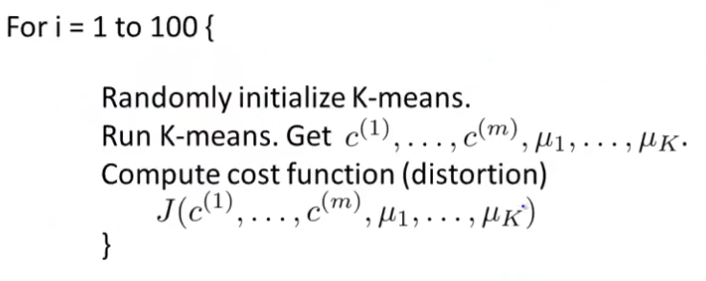Here you can change the iteration number from 100 to anything you like, just make sure you get the cluster with the minimum cost without compromising the time complexity for the iterations.

This pretty much sums up the K-means clustering algorithm. Don't forget to leave a comment below and let me know if this post came in any help to you. And of course feel free to ask anything you like to know and tell me what you didn't understand.

Until next time. Happy learning.